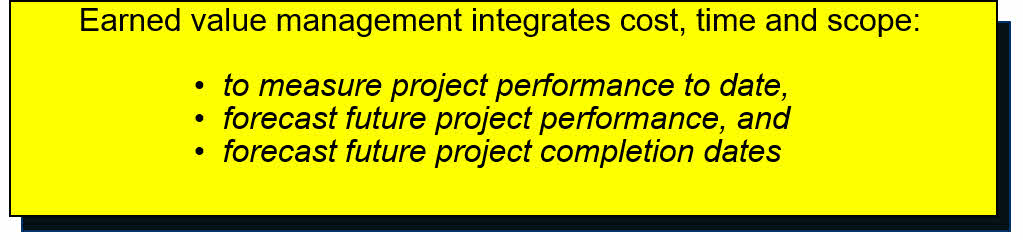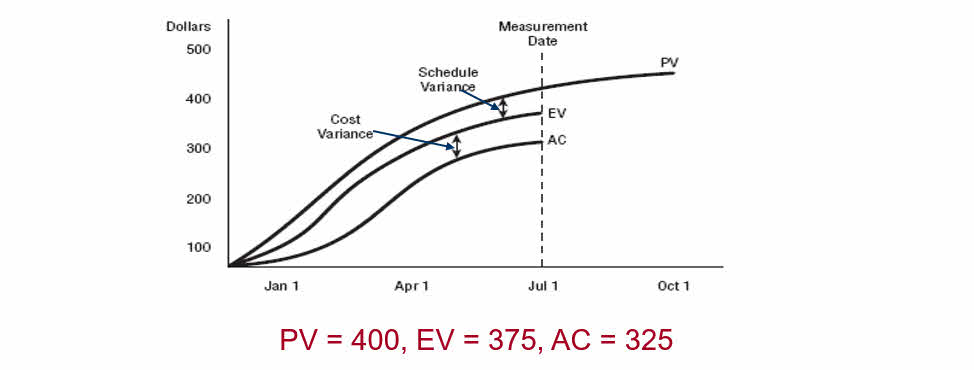## Basic Concepts of Earned Value Management (EVM)- Part 1

Many project managers manage their project performance by comparing planned to actual results. With this approach, you could easily be on time but overspend according to your plan.

A better method is Earned Value Management (EVM). Simply stated, EMV compares what you’ve received or produced to what you’ve spent.The EVM continuously monitors the percent complete of the project, the planned value (PV), earned value (EV), and actual costs (AC) expended to produce the work of the project. When variances that result in cost changes are discovered (including schedule variances and cost variances), those changes are managed using the cost change control system.

The primary function of this analysis technique is to:

• determine and document the cause of the variance,
• to determine the impact of the variance (you’ll do this with the EVM formulas shortly), and
• to determine whether a corrective action should be implemented as a result.

To perform the EVM calculations, you need to first gather the four measurements mentioned earlier: the percent complete of the project, the planned value (PV), actual cost (AC), and earned value (EV).

## Percent complete of project

Many project managers determine how much work has been completed by asking team members for an estimate of percent complete for each task. On projects where work cannot be measured, this estimate is simply a guess. This is time consuming and almost always a complete waste of time because a guess does not provide a confident estimate of the actual percent complete.

If a project has been planned using a WBS, and tasks require about 80 hours of work, we have alternatives to percent complete.

Because tasks will be completed faster and more frequently, we can forget percent complete and use one of the following:

• 50/50 RULE – A task is considered 50% complete when it starts. The remaining 50% credit is given when the task is completed
• 20/80 RULE – A task is considered 20% complete when it starts. The remaining 80% credit is given when the task is completed
• 0/100 RULE – A task does not get credit for partial completion, it get 100% credit only full completion.

## Planned value (PV)

The planned value (PV) is the cost of work that has been approved (budgeted) for a schedule activity or WBS component to be completed during a given time period. These budgets are established during the planning processes.

The total PV is also referred as “Budget at Completion” (BAC), and/or “Performance Measurement Baseline” (PMB), PV is also called budgeted cost of work scheduled (BCWS).

Example:

• According to my project plan By July , I plan to complete several activities that have been approved (budgeted) at \$ 400.
• Therefore at the end of the first month the planned value PV = \$ 400.

## Earned value

Earned value (EV) is the value of the work (schedule activity or WBS component) completed to date as it compares to the budgeted amount (PV) assigned to the work component. EV is also called budgeted cost of work performed (BCWP).

• EV cannot be greater than PV

EV = PV * percent complete

Example:

• Suppose that at July 1, I completed only 81% of my planned activities
• Therefore at July 1,    EV = \$ 400 * 81% = \$ 325

## Actual cost

Actual cost (AC) is the cost of completing the work (a schedule activity or WBS component) in a given time period. AC is also called actual cost of work performed (ACWP).

Actual costs might include direct and indirect costs but must correspond to what was budgeted for the activity.

• If the budgeted amount did not include indirect costs, do not include them here

Later you’ll see how to compare this to PV to come up with variance calculation results.

Example:

• Suppose that at July 1, I collect all the actual costs and the total figure is \$ 325.
• Therefore at the end of the first month AC = \$325

We can plot all the PV, AC, and EV measurements graphically to show the variances between them.

• If there are no variances in the measurements, all the lines on the graph remain the same, which means the project is progressing as planned.

The following figure shows an example that plots these three measurements## Cost Variance

Cost variance is one of the most popular variances that project managers use. Cost variance shows whether your actual costs are higher than budgeted (with a resulting negative number) or lower than budgeted (with a resulting positive number).

The cost variance (CV) is calculated as follows:

CV = EV – AC

• CV = Negative, OVER BUDGET
• CV = Positive, UNDER BUDGET

In our Example

• CV = \$ 375-\$ 345 = \$ 50 (\$50 under budget as of July 1)
• A negative cost variance is often non-recoverable

## Schedule Variance

Schedule variance, also a popular variance, tells you whether the schedule is ahead or behind what was planned for this period in time. The schedule variance (SV) is calculated as follows:

SV = EV – PV

• SV = Negative, BEHIND SCHEDULE
• SV = Positive, AHEAD OF SCHEDULE

Lets plug in the numbers:

• SV = \$375-\$400 = -\$25 (Behind schedule as of July 1)

# Performance Indexes

Together, the CV and SV are known as efficiency indicators for the project.

Cost and schedule performance indexes, (CPI and SPI) are primarily used to calculate performance efficiencies. They’re often used in trend analysis to predict future performance.

You’ll need to know the calculations and what the results mean.

• If CPI or SPI is greater than 1, you’ve got better than expected performance. If the result is less than 1, you’ve got poor performance. If it equals 1, you’re right on target.

## Cost Performance Index (CPI)

The cost performance index (CPI) is calculated this way:

• CPI = EV ÷ AC

Let’s plug in the numbers:

• CPI = 375 ÷ 325 =1.15
• Interpretation: as of July 1, we are getting \$1.15 for every dollar invested on this project

## Schedule Performance Index (SPI)

The schedule performance index (SPI) is calculated this way:

• SPI = EV ÷ PV

Let’s plug in the numbers:

• SPI = 375 ÷ 400 =0.94
• Interpretation: Uh-oh, not so good. You are only progressing at 94% of the rate planned

In the next article we will discuss the Forecasting